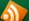# Swimming Pool News, Hints & TipsApril
22
2010

# How do I figure the amount of water in my pool?

To determine pool water volume (total gallons), multiply (in feet) the Length x Width x Average Depth x 7.5 = Total Gallons. Average depth, is the depth of the shallow end added to the depth of the deep end, and then divided in half. The 7.5 figure represents the total volume of water in a cubic foot. An example for an average pool would be:  15′ x 30′ x 6′ x 7.5 = 20,250 Total Gallons. Note: Having an accurate pool water volume is important to ensure that all dosage recommendations are correct.